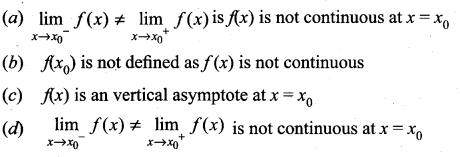## Tamilnadu Samacheer Kalvi 11th Maths Solutions Chapter 9 Limits and Continuity Ex 9.5

Question 1.
Prove that f(x) = 2x2 + 3x – 5 is continuous at all points in R.
Solution:
Polynomial functions are continuous at every points of R.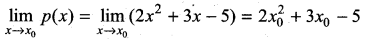Question 2.
Examine the continuity of the following:
(i) x + sin x
Solution:
f(x) = x + sin x
The Domain of the function (-∞, ∞)
∴ f(x) is continuous in (-∞, ∞)(i.e.,) for all x ∈ R

(ii) x2 cos x
Solution:
f(x) = x2 cos x
The Domain of the function (-∞, ∞)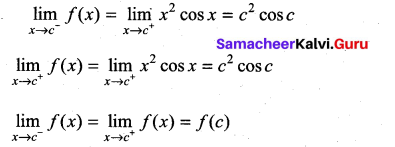f(x) is continuous in R

(iii) ex tan x
Solution:
The Domain of the function in R – {(2n + 1) π/2}
∴ The functions is continuous for all x ∈ R – (2n + 1) $$\frac{\pi}{2}$$, n ∈ Z

(iv) e2x + x2
f(x) = e2x + x2 = 1 + 2x + $$\frac{(2 x)^{2}}{2 !}$$ + …………. + x2
Solution:
∴ The functions is continuous for all x ∈ R

(v) x.ln x
Solution:
Thus f(x) is continuous for (0, ∞)

(vi) $$\frac{\sin x}{x^{2}}$$
Solution:
Thus f(x) is continuous for all x ∈ R – {0}

(vii) $$\frac{x^{2}-16}{x+4}$$
Solution:
f(x) = $$\frac{x^{2}-16}{x+4}=\frac{(x-4)(x+4)}{x+4}$$
The function f(x) is continuous for all x ∈ R – {-4}

(viii) |x + 2| + |x – 1|
Solution:
f(x) is continuous for x ∈ R

(ix) $$\frac{|x-2|}{|x+1|}$$
Solution:
The function is continuous for all x ∈ R – {-1}

(x) cot x + tan x
Solution:
The function is continuous for all x ∈ R – $$\frac{n \pi}{2}$$, n ∈ z.Question 3.
Find the points of discontinuity of the function f, where,
(i)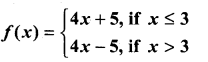Solution:
f(3) = 12 + 5 = 17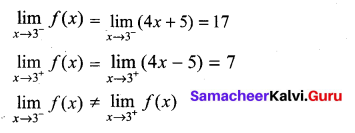∴ f(x) is discontinuous at x = 3

(ii)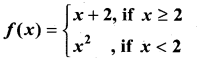Solution:
f(x) = 4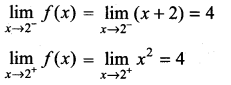∴ f(x) is continuous for all x ∈ R

(iii)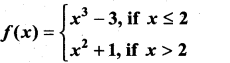Solution:
f(x) = 8 – 3 = 5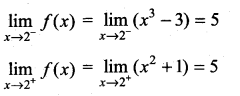∴ f(x) is continuous for all x ∈ R

(iv)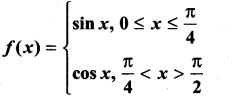Solution: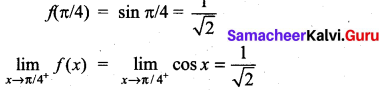∴ f(x) is continuous for all x ∈ [0, π/2]Question 4.
At the given points x0 discover whether the given function is continuous or discontinuous citing the reasons for your answer.
(i)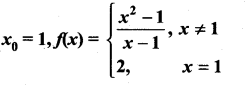Solution:
Given f(x0) = 1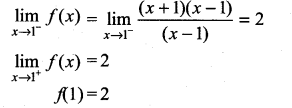∴ f(x) is continuous at x0 = 1

(ii)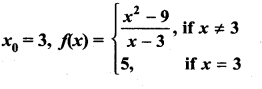Solution: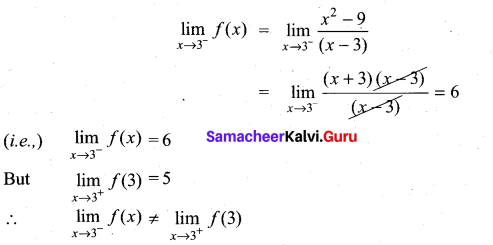∴ f(x) is not continuous at x0 = 3

Question 5.
Show that the function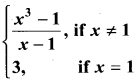is continuous on (-∞, ∞)
Solution: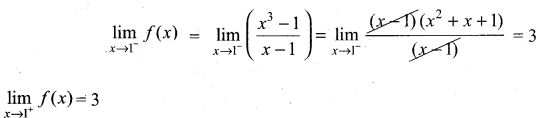Given that f(1) = 3
∴ f(x) is continuous for all x ∈ R

Question 6.
For what value of α is this function f(x) =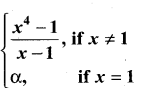continuous at x = 1?
Solution: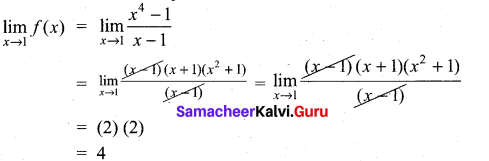∵ f(x) is continuous at x = 1, α = 4

Question 7.
Let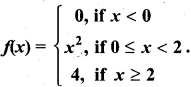Graph the function. Show that f(x) continuous on (-∞, ∞)
Solution: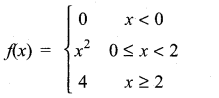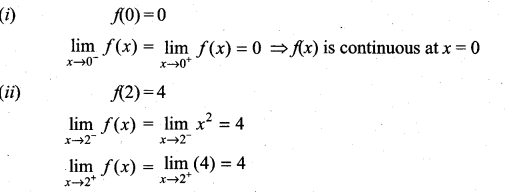∴ f(x) is continuous in (-∞, ∞)

Question 8.
If f and g are continuous function with f(3) = 5 and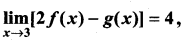find g(3).
Solution:
Since f and g are continuous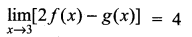2f(3) – g(3) = 4
2(5) – g(3) = 4
10 – 4 = g(3)
g(3) = 6Question 9.
Find the points at which f is discontinuous. At which of these points f is continuous from the right, from the left, or neither? Sketch the graph of f.
(i)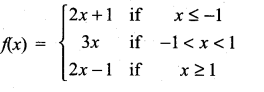∴ f(x) is not continuous at x = 1
Solution: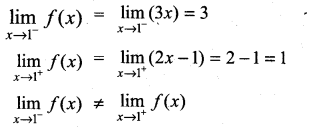f(x) is not continuous at x = 1

(ii)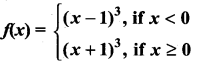Solution: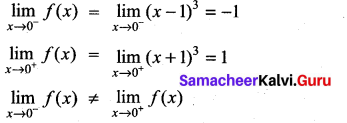∴ f(x) is not continuous at x = 0

Question 10.
A function f is defined as follows: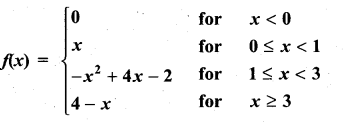Is the function continuous?
Solution: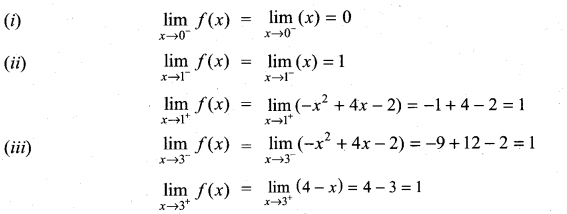From (i), (ii) and (iii)
f(x) is continuous at x = 0, 1, 3

Question 11.
Which of the following functions f has removable discontinuity at x = x0? If the discontinuity is removable, find a function g that agrees with f for x ≠ x0 and is continuous on R.
(i) f(x) = $$\frac{x^{2}-2 x-8}{x+2}$$, x0 = -2
Solution: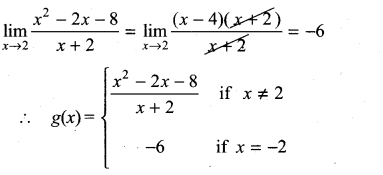(ii) f(x) = $$\frac{x^{3}+64}{x+4}$$, x0 = -4
Solution: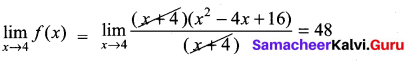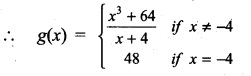(iii) f(x) = $$\frac{3-\sqrt{x}}{9-x}$$, x0 = 9
Solution: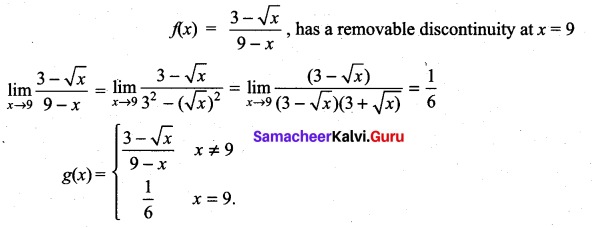Question 12.
Find the constant b that makes g continuous on (-∞, ∞)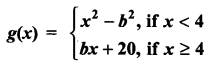Solution:
Since g(x) is continuous,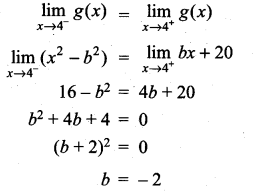Question 13.
Consider the function f(x) = x sin $$\frac{\pi}{x}$$. What value must we give f(0) in order to make the function continuous everywhere?
Solution: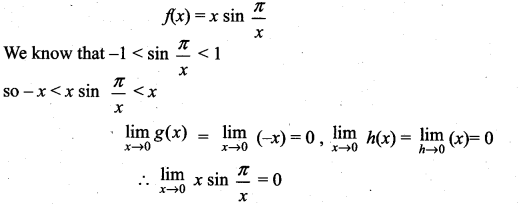so to make the function f(x) is continuous at f(0) = 0

Question 14.
The function f(x) = $$\frac{x^{2}-1}{x^{3}-1}$$ is not defined at x = 1. What value must we give f(1) in order to make f(x) continuous at x = 1?
Solution: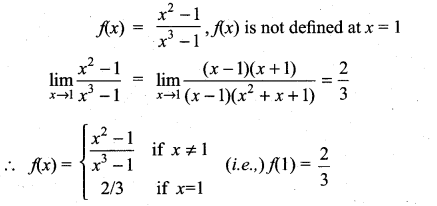Question 15.
State how continuity is destroyed at x = x0 for each of the following graphs.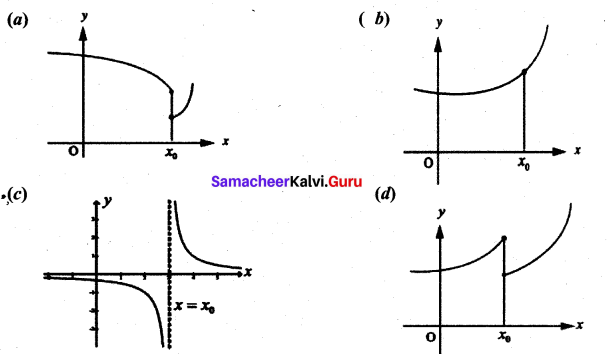Solution: